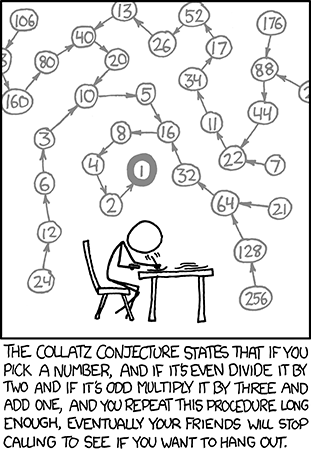Too Good To Miss: Terry Tao Almost Proves Collatz Conjecture
Written by Mike James
Tuesday, 31 December 2019

There are some news items from the past year that deserve a second chance. Here we have one such - although not as well known as the long standing P=NP conjecture, Collatz has fascinated people for the past eight decades and produced almost as many flawed proofs. Now mathematician Terence Tao seems to be close to a proof.

(2019-09-13)

If you don't know what the Collatz conjecture is then you haven't missed out on anything really important - with the possible exception of the excellent xkcd cartoon reproduced below:

####The cartoon is accurate, but let's make the conjecture, which was proposed in 1937 by German mathematician Lothar Collatz, clear:

• Pick a number, a positive integer
• If even divide by 2
• If odd multiply by 3 and add one
• Repeat above two steps with new value

If you try it you will discover that you eventually reach a result of 1. For example, 10, 5,16, 8, 4, 2, 1. At first you think it must be an accident and so you try a few more tests. Then you become obsessed and write a program - and you always end up at 1.

So far it has been verified for values up to 5.76x10^18.

The Collatz conjecture is that this is indeed always true, but can you prove it?

If you think it should be easy then it is worth knowning that Paul Erdős proclaimed:

"Mathematics may not be ready for such problems"

Terence Tao at the University of Califonia is closer to a proof than we have ever been. Why isn't it a proof? Because it's a probability argument:

Almost all orbits of the Collatz map attain almost bounded values.

Why is this important? The reason is that if you can show that the minimum of the Collatz sequence for N is always smaller N for all N>1 then you have proved the Collatz conjecture. The reason is simple. If you get a value m which is smaller than N then the rest of the sequence for N is the same as that as if you had started from m and so we now have that the minimum for the sequence is less than m. You can repeat this and work back to prove the the minimum has to be 1.

Tao hasn't quite proved this but he has proved (rewritten to be closer to English):

Theorem 2  Let f be any function defined on the integers, excluding zero, and f(N) goes to infinity with N  then the minimum of the Collatz sequence for N is less than f(N) for almost all N.

If you take f to be the identity than we have the minimum Collatz value for N is smaller than N.

So problem solved?

Not quite. The weasel word in the theorem is "for almost all". This is a signal that the theorem is probablistic. What this means is that the set of N for which this is true is dense (in the sense of logarithmic density). What this means, in a non-technical sense is that we have proved that the theorem is true for all but an insignificant number of cases.

An insignificant nubmer of cases also includes no cases at all, so the theorem might apply to all N or there might a few examples where it doesn't apply and the Collatz conjecture is wrong after all.

So which is it?

As Tao writes in an answer to a comment to his blog post:

"...but this is one of these situations where there seems to be an enormous gap in difficulty between “almost all” results and “all” results."

It looks as if the Collatz conjecture is going to hold out for a while longer. Armed with this probablistic argument it might be possible to see new reasons why it is true.

Almost all orbits of the Collatz map attain almost bounded values (paper pdf)

#### Related Articles

Erdos Conjecture Proven

Travelling Salesman - A Movie About P=NP

Collatz conjecture proved?

Update on the Proof of P≠NP?

NP-Complete - Why So Hard?

Computational Complexity

Computability

 Nvidia Releases Updated AI Framework02/08/2022NVIDIA has announced the general availability of NVIDIA AI Enterprise 2.1. The improvements to the AI and data analytics software include new support for containers, and for public clouds. + Full Story RoboCup 2022 - What's The Score?22/07/2022This year's RoboCup took place in Bangkok, Thailand and attracted about 1,400 participants from 39 countries and regions who compete against each other in 17 disciplines. + Full Story More News